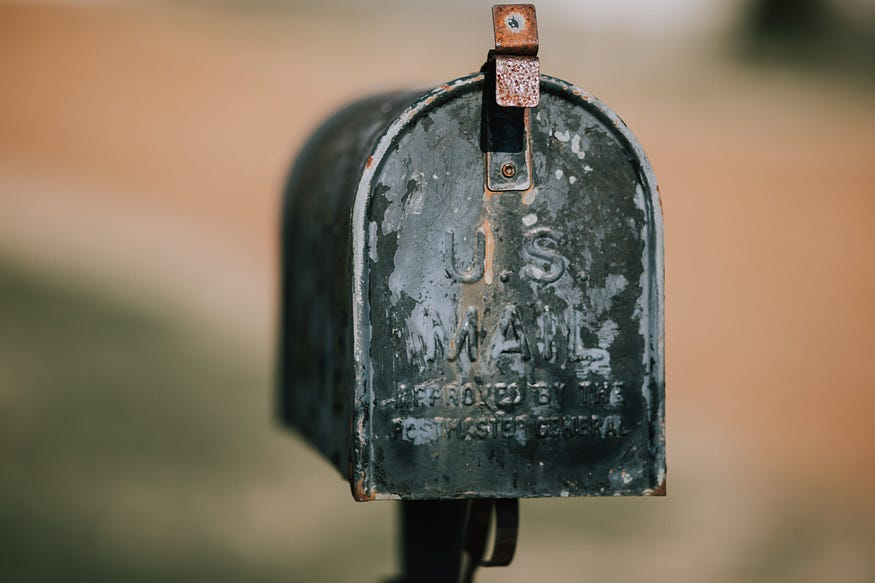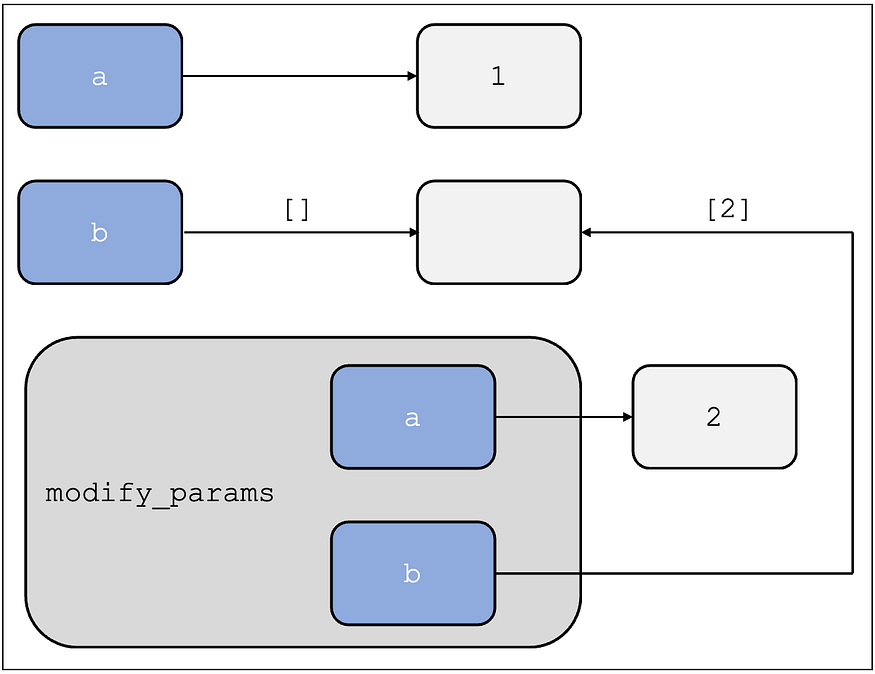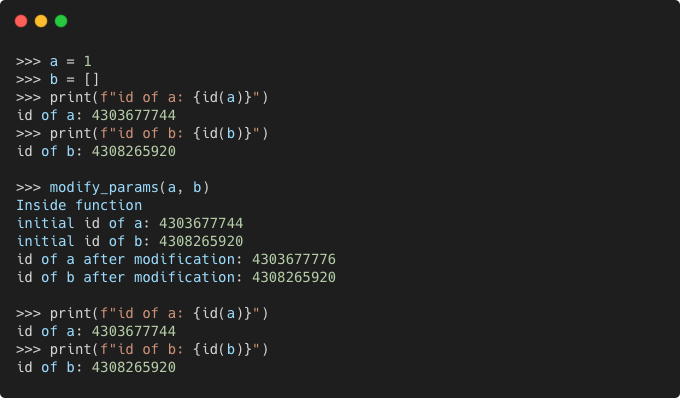# Pass By Object Reference in Python

## Understanding “pass by object reference” in Python.When I first encountered an example of a function modifying an integer parameter being passed to it in Python, I was surprised that the value was unchanged in the caller, outside the function scope. What perplexed me, even more, was that for lists, the value did in fact change in the caller!

Let us look at a simple Python code to demonstrate this behavior:

``````def modify_params(a: int, b: List[int]) -> None:
"""
Increments `a` and appends `a` to list `b`.
"""
a += 1
b.append(a)

>>> a = 1
>>> b = []
>>> a, b
(1, [])
>>> modify_params(a, b)
>>> a, b
(1, )
``````

Python Parameter Modification Example

Upon digging a bit more into this seemingly bizarre behavior, I learned that this is because of the “Pass By Object Reference” parameter passing scheme used by Python.

Two of the more common parameter passing schemes used by programming languages are:

1. Pass By Value, where a copy of the original value is passed as a parameter and hence the original value in the caller remains unchanged.
2. Pass By Reference, where a reference to the original value is passed as the parameter and so the original value in the caller is also modified.

In Python’s Pass By Object Reference, the object reference is passed by value. A variable can be thought of as a name (or reference) assigned to an object, and the object can be mutable (eg. list) or immutable (eg. integer). When a variable is passed as a function parameter, actually a copy of this reference is passed.Pass-By-Object-Reference for Mutable and Immutable Types

Now when we modify this reference within the function, the referenced object is modified for the mutable objects, but the reference copy just references a new object for the immutable objects.

Let us update the `modify_params` function in the above code snippet to print the `id` of the parameters before and after their modification inside the function:

``````def modify_params(a: int, b: List[int]) -> None:
"""
Increments `a` and appends `a` to list `b`.
"""
print("Inside function")

print(f"initial id of a: {id(a)}")
print(f"initial id of b: {id(b)}")

a += 1
b.append(a)

print(f"id of a after modification: {id(a)}")
print(f"id of b after modification: {id(b)}")
``````

Updated Function to Print Parameter IDsVariable IDs in the Caller and within the Function

As we can observe, the variables in the caller reference the same objects before and after the function `modify_params` is called.

Within the `modify_params` function, the parameters reference the same objects as they do in the caller before the variables are modified. After the variables are modified, the variable `a` referencing the immutable object `int`, references a new object, but the variable `b` referencing the mutable object `list`, still references the old object and it gets modified.

I hope this helps clarify the parameter passing scheme used in Python and avoid certain confounding bugs!